# js作用域详解

## js对象

``````var a = 1;
console.log
``````

``````interface Number {
/**
* Returns a string representation of an object.
* @param radix Specifies a radix for converting numeric values to strings. This value is only used for numbers.
*/

/**
* Returns a string representing a number in fixed-point notation.
* @param fractionDigits Number of digits after the decimal point. Must be in the range 0 - 20, inclusive.
*/
toFixed(fractionDigits?: number): string;

/**
* Returns a string containing a number represented in exponential notation.
* @param fractionDigits Number of digits after the decimal point. Must be in the range 0 - 20, inclusive.
*/
toExponential(fractionDigits?: number): string;

/**
* Returns a string containing a number represented either in exponential or fixed-point notation with a specified number of digits.
* @param precision Number of significant digits. Must be in the range 1 - 21, inclusive.
*/
toPrecision(precision?: number): string;

/** Returns the primitive value of the specified object. */
valueOf(): number;
}
``````

``````function atest() {
console.log(1);
}
``````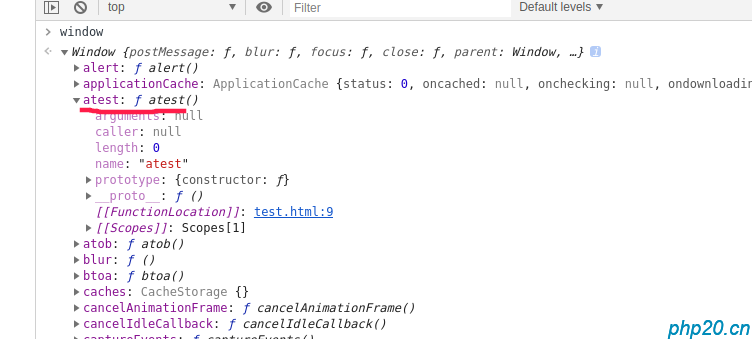atest其实是一个window对象下的方法对象

## var 局部变量作用域

### var 声明一个对象，只作用域当前作用域以下。例如：

``````var a = 'test';
function b() {
console.log(a);
}
b();
``````

b函数可以成功调用上层作用域变量：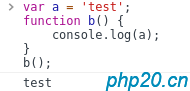### 但是不能被上层作用域调用：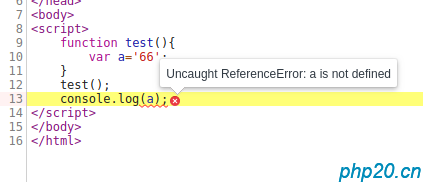### 在顶级作用域下，var 声明变量，等于全局变量，被window对象接管:

``````<script>
var aaaa='666';
</script>
``````## 定义全局作用域变量

``````<script>
var  a1='666';
a2='777';
function test() {
a3='888';
}
test();
console.log(a1,a2,a3);
</script>
``````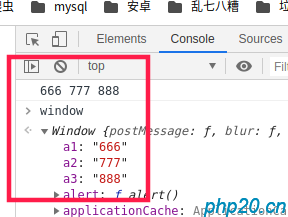## window对象直接赋值

window对象直接赋值方法，等同于不使用var 关键字定义：

``````<script>
window.a1='666';
window.a2='777';
function test() {
a3='888';
}
test();
console.log(a1,a2,a3);
</script>
``````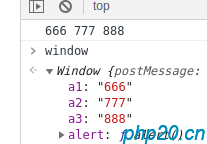``````<script>
window.test = function () {
console.log(666);
};
test();
</script>
``````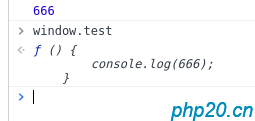## 变量作用域覆盖问题

``````<script>
var a=1;
function atest() {
btest();
function btest() {
var a=2;
console.log('btest:'+a);
}
console.log("atest："+a);
}
atest();
</script>
``````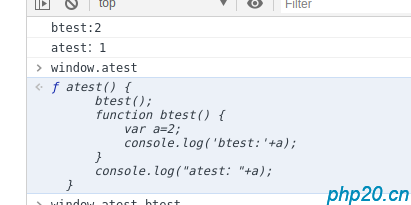## 闭包函数，闭包作用域

### 闭包函数，又称匿名函数，例如：

``````<script>
(function () {
var a = 1;
console.log(a);
});
console.log(a);
</script>
``````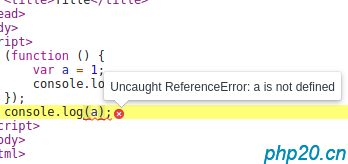``````<script>
(function () {
var a = 1;
console.log(a);
})();
console.log(a);
</script>
``````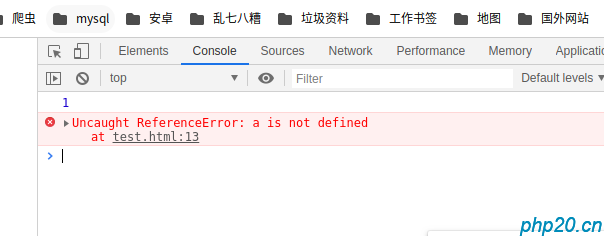### 赋值闭包函数

``````<script>
var atest = function () {
console.log('hello')
};
atest();
atest();
</script>
``````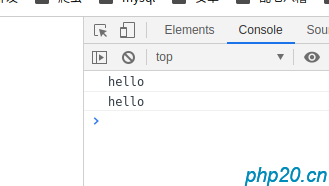``````<script>
var atest = function () {
var a='666';
console.log('hello');
return a;
}();
console.log(atest);
</script>
``````## 闭包返回对象

``````<script>
var atest = function () {
var a=1;
return{
a:2,
b:3,
c:function () {
//返回上层闭包声明的a变量
return a;
}
};
}();
console.log(atest.c());
console.log(atest.a);//输出闭包return的json对象属性
console.log(atest.b);
</script>
``````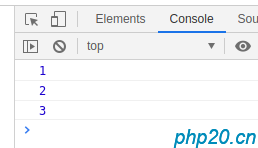return作用域变量访问情况

``````<script>
var atest = function () {
return{
a:2,
b:1,
getA:function () {
//返回上层闭包声明的a变量
return this.a;
},
getB:function () {
//返回上层闭包声明的a变量
return this.b;
}
};
}();
console.log(atest.getA());
console.log(atest.getB());
atest.a=7;
atest.b=6;
console.log(atest.getA());
console.log(atest.getB());
</script>
``````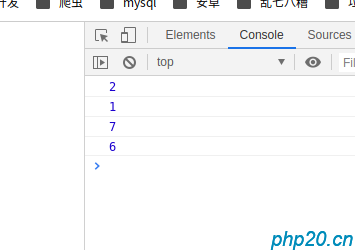# 总结

1：js万物皆对象，所有变量都是对象类型。

2：js的作用域是往下通用的，下层作用域可访问上层作用域的变量，并可修改值

3：js下层作用域变量和上层同名冲突时，下层作用域将覆盖上层变量，但上层作用域的访问不受影响

4：不适用var方法定义的变量，都属于全局变量，也就是window对象的属性

5：可通过window.方法定义全局变量

6：在顶层作用域声明的函数，其实就是window对象的方法

7：闭包在首次声明时，需要加括号自动调用，否则不能调用

8：闭包函数可通过赋值方法调用

9：闭包函数加括号 赋值到变量中，该变量将等于闭包的返回值

10：闭包返回值可返回一个对象，后期可使用  闭包赋值变量名.对象属性方法进行访问

11：闭包函数类var 的变量，只能在闭包函数下层或当前层使用，外部无法访问，但可以通过return 对象中，声明一个方法进行访问

12：闭包函数return的对象，都可以被  外部通过闭包赋值变量名.对象属性 应用，而对象本身也可以通过this.变量名，方式应用

##### 热门推荐
•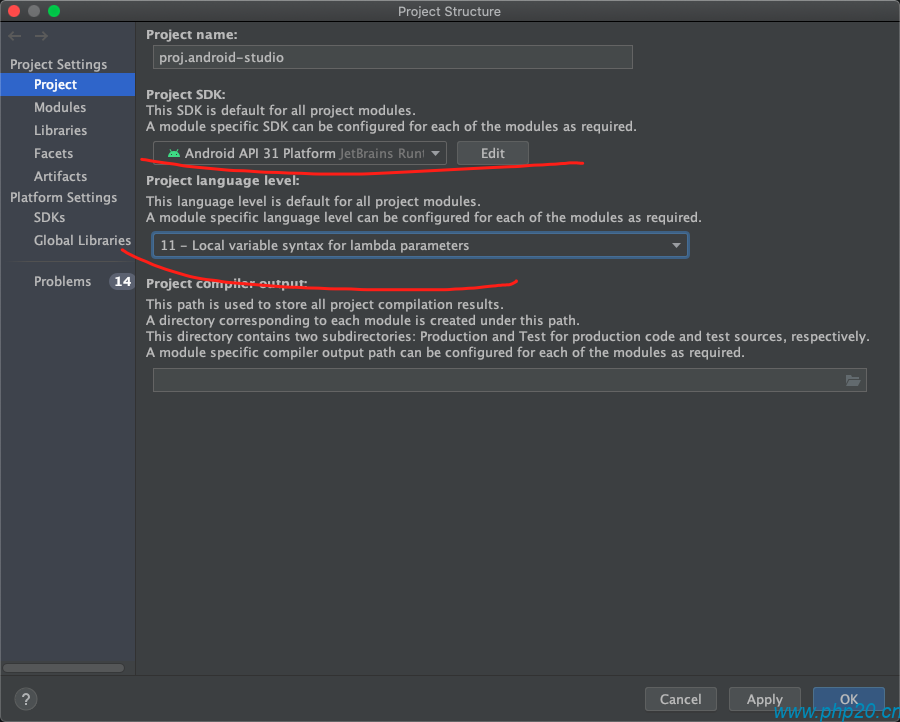浏览(2,242)
•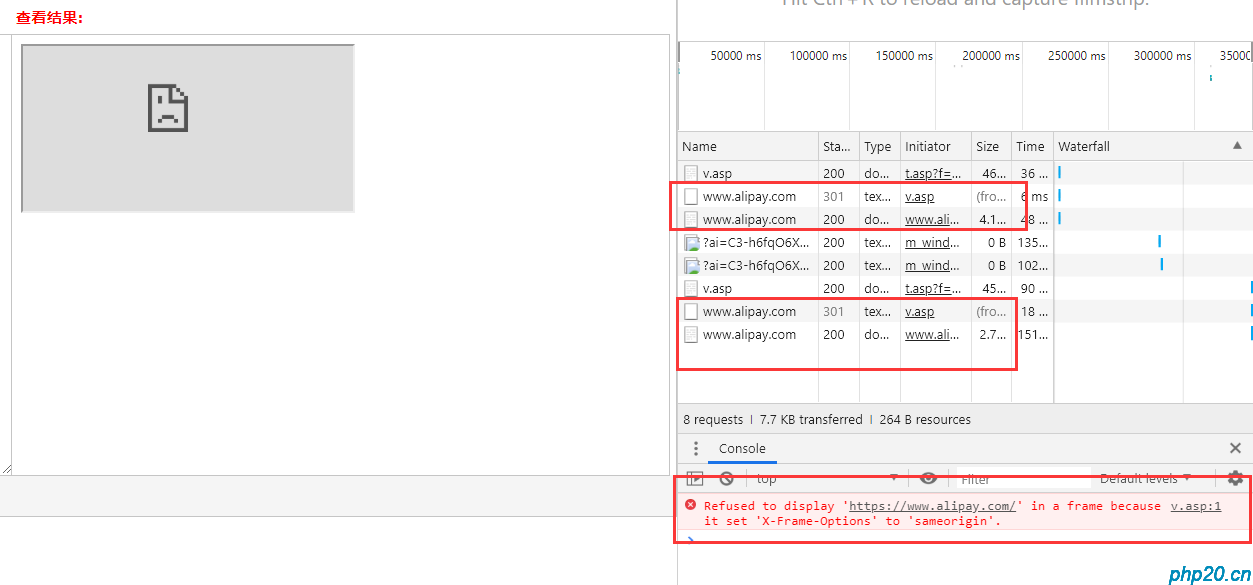浏览(1,298)
••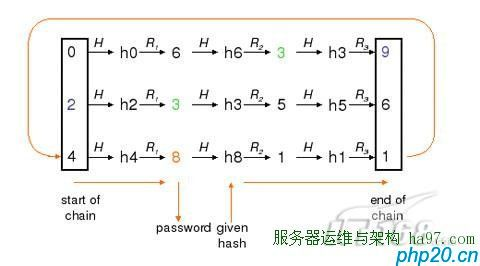•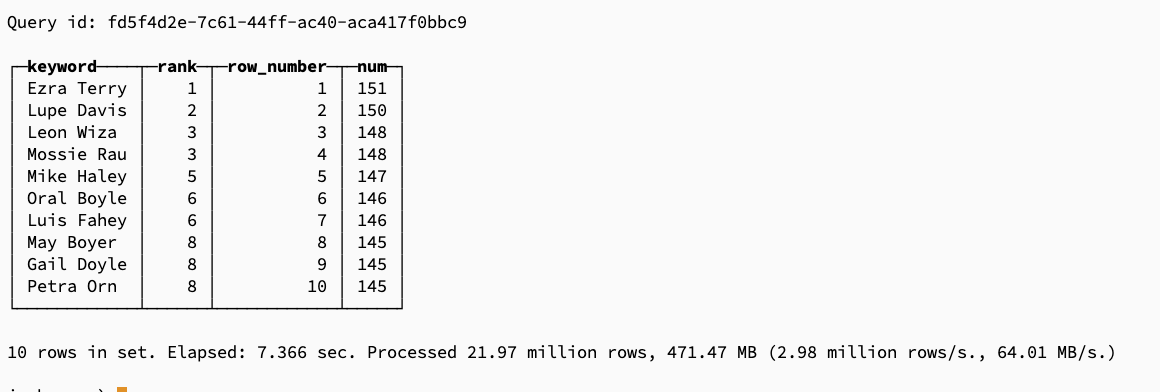浏览(1,011)
•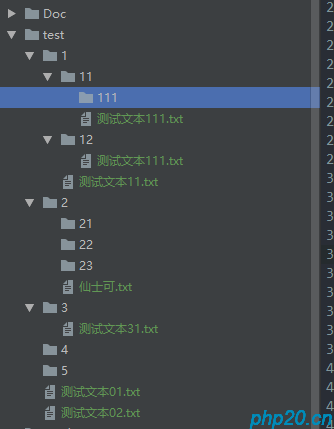浏览(965)
•浏览(915)
•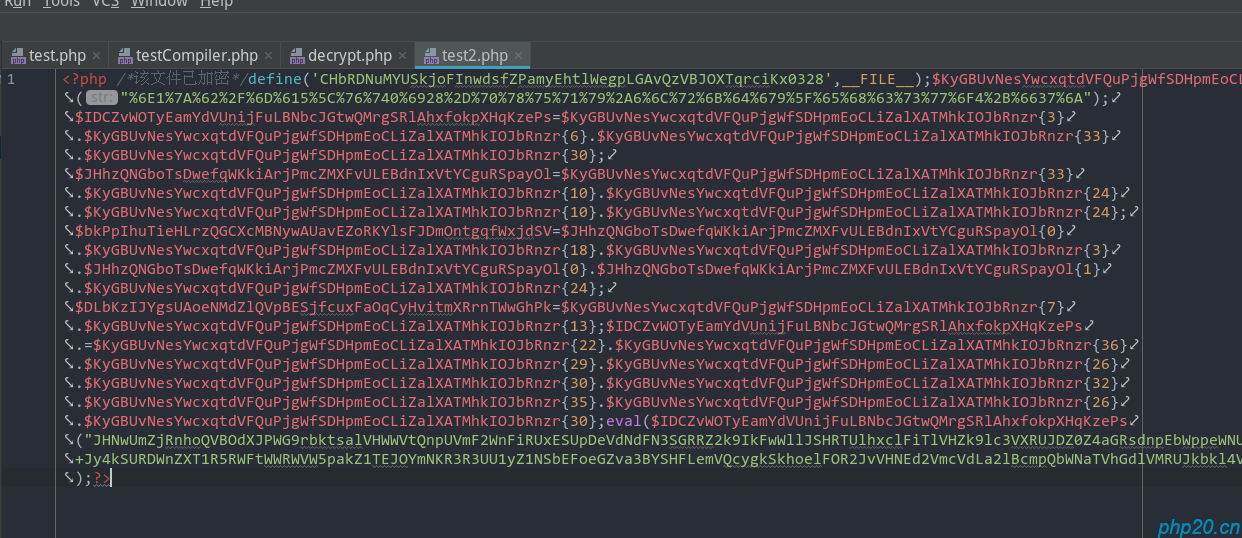浏览(864)
•浏览(842)
•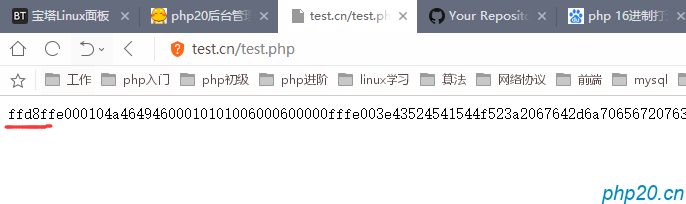浏览(735)